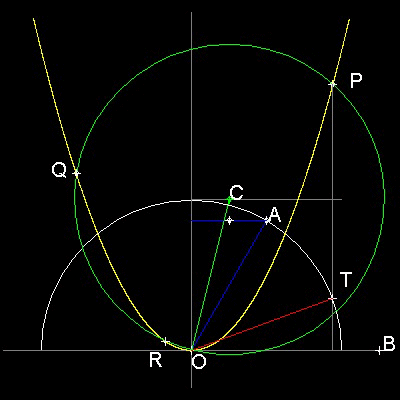Special Curves
Go to   Fun_Math Content Table   Trisecting an Angle   Special Curves

### Trisection using Special Curves

#### Parabola by Rene Descartes

Rene Descartes (1596 - 1650) showed that Angle Trisection can be done by using Parabola. His idea is shown in the figure shown below.

You can see the process in animation.##### La Geometrie by Rene Descartes
Quote from The Preface of the English translation of Rene Descartes La Geometrie: "If a mathematician were asked to name the great epoch-making works in his science,he might well hesitate in his decision concering the product of the nineteenth century; he might even hesitate with respect to the eighteenth century; but as to the product of the sixteenth and seventeenth centuries, and particularly as to the works of the Greeks in classical times,he would have very definite views. He would certainly include the works of Euclid, Archimedes, and Apollonius among the products of the Greek civilization, which among those which contributed to the great renaissance of mathematics in the seventeenth century, he would as certainly include La Geometrie of Descartes and the Principia of Newton"
******** parabola_tri_desc.dwg ********

He showed that both Delian and Trisection problems are equivalent to solving the cubic equations, and its solution are obtained by using conics( parabola is one of them. )

In the case of trisection, the roots of the trisection equation: x3 - 3x -2a = 0 , where a = cos(3q) are represented by the x-coordinate values of intersection of (1) parabola and (2) a circle with its center at point (a, 2).Thus,

(1) y = x2

(2) x2 + y2 - 2ax - 4y = 0

By substituting (1) into (2) ,y is eliminated and the resulting equation only for x is

x ( x3 - 3x -2a ) = 0 , or x3 - 3x -2a = 0

To create this drawing and animation:
Then from command line, type parabola_trisection

This will let users define angle to be trisected, draw parabola and circle. The rest of the operaration will be done manually.
Example: AOB = 60 degrees case1. Input 2<60. to specify point A
2. Drop a line (blue) perpendicular to y-axis. mark the mid point.
3. Find point C on the line y=1 drawing a line parallel line through the mid point.
4. With the center at C, draw a circle (green) with radius CO.
5. Intersection points between green circle and yellow parabola are P, Q & R.
6. Drop a line from point P perpendicular to x-axis.
7. This line intersects the unit circle at point T.
8. angle TOB trisects angle AOB.
Question: How about points Q & R ?
** parabola_trisection_60_deg_case.dwg **

Go to   Fun_Math Content Table   Trisecting an Angle   Special Curves

All questions/suggestions should be sent to Takaya Iwamoto

Last Updated Nov 22, 2006